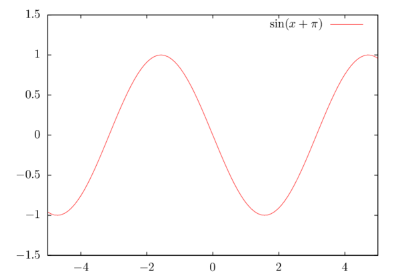Categories

# LaTeX formulas in gnuplot

As a reminder to myself: On the TeX Stackexchange, there is a nice question and discussion by me and some helpful people on how to use LaTeX code in gnuplot / how to embed LaTeX equations in a plot. A copy of my revised answer:

First, we set up a gnuplot called test.plt:

plot [-5:5] [-1.5:1.5] sin(x+pi) title "$\sin(x+\pi)$"


Then we also set up a small Makefile:

.SUFFIXES: .plt .tex .pdf

%.tex: %.plt
gnuplot -e "
set format '$%g$' ;
set terminal epslatex standalone color ;
set output '$@' "$<

%.pdf: %.tex
pdflatex \$<

all: test.pdf


Running “make all” will produce this plot: